# GMAT Math : Calculating the area of a right triangle

## Example Questions

### Example Question #21 : Triangles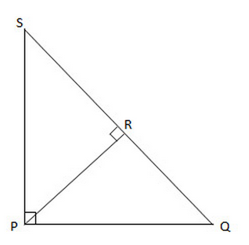In the above figure, triangle SPQ, SPR and PRQ are right triangles. Given the lengths of SP and PQ are 2cm. What is the area of triangle SPR?Explanation:

Sinceare right triangles, we know that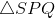is an isosceles right triangle. So we know that the lengths of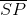and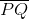are 2 cm, so we can get the length of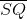by using the Pythagorean Theorem: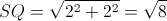is the midpoint of, so the length of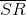is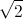.

Now we can use the Pythagorean Theorem again to solve for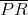: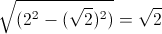.

Finally, we have all the elements needed to solve for the area of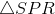: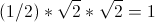### Example Question #22 : Triangles

If a right triangle has a hypotenuse that is 10, and one side is 6, find the area of the triangle.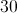Explanation:

Becaue this is a right triangle and since the hypotenuse is 10 and one side is 6, the other side will be 8.  This can be found using the Pythagorean Theorem, or multiplying a 3-4-5 triangle by 2 to get a 6-8-10 triangle.  Since the area of a right triangle is half of the product of the two sides, we have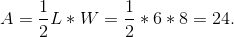### Example Question #23 : Triangles

The hypotenuse of a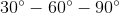triangle is equal to the sidelength of a square. Give the ratio of the area of the square to that of the triangle.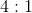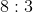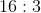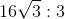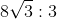Explanation:

Letbe the sidelength of the square. Then its area is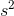.

If the hypotenuse of atriangle is, its shorter leg is half that, or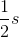; its longer leg is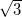times the shorter leg, or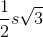. The area of the triangle is half the product of the legs, or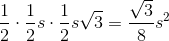The ratio of the area of the square to that of the triangle is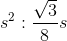or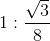or### Example Question #24 : Triangles

A triangle on the coordinate plane has vertices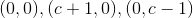.

Which of the following expressions is equal to the area of the triangle?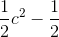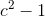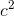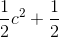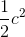Explanation:

This is a right triangle with legs along the- and-axes, so the area of each can be calculated by taking one-half the product of the two legs.

The vertical leg has length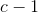; the horizontal leg has length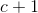.

Now calculate the area: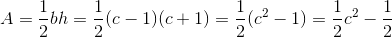### Example Question #31 : Triangles

Calculate the area of the following right triangle, leave in terms of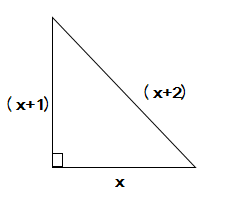(Not drawn to scale.)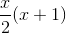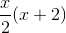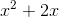Explanation:

The equation for the area of a right triangle is: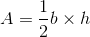In this case, our values are: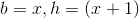Plugging this into the equation leaves us with: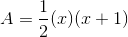which can be rewritten as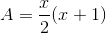### Example Question #32 : Triangles

A right triangle has a hypotenuse of 13 and a base of 12. Calculate the area of the triangle.Explanation:

In order to calculate the area of a right triangle, we need to know the height and the base. We are only given the length of the base, so we first need to use the Pythagorean theorem with the given hypotenuse to find the length of the height: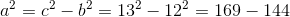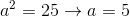Now that we have the height and the base, we can plug these values into the formula for the area of a right triangle: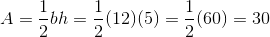### Example Question #33 : Triangles

Find the area of a triangle whose base isand height is.Explanation:

To find the area, use the following formula: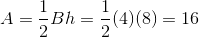Tired of practice problems?

Try live online GMAT prep today.